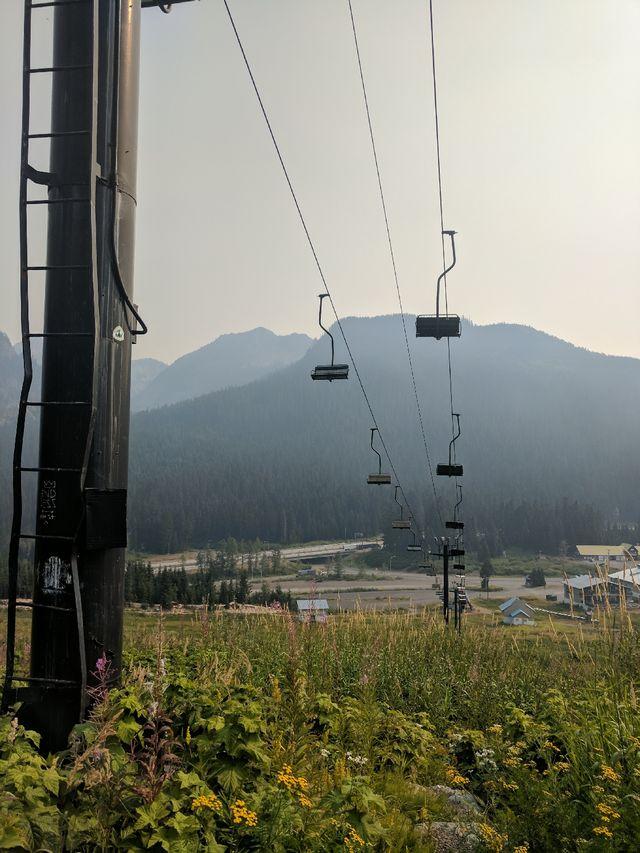# PCTself.__wrap_b=(t,n,e)=>{e=e||document.querySelector(`[data-br="\${t}"]`);let s=e.parentElement,r=R=>e.style.maxWidth=R+"px";e.style.maxWidth="";let o=s.clientWidth,i=s.clientHeight,c=o/2-.25,l=o+.5,u;if(o){for(;c+1<l;)u=Math.round((c+l)/2),r(u),s.clientHeight===i?l=u:c=u;r(l*n+o*(1-n))}e.__wrap_o||(e.__wrap_o=new ResizeObserver(()=>{self.__wrap_b(0,+e.dataset.brr,e)})).observe(s)};self.__wrap_b(":Rab6:",1)

Washington: ups and downsAug 27 2017

# Snoqualmie Passself.__wrap_b=(t,n,e)=>{e=e||document.querySelector(`[data-br="\${t}"]`);let s=e.parentElement,r=R=>e.style.maxWidth=R+"px";e.style.maxWidth="";let o=s.clientWidth,i=s.clientHeight,c=o/2-.25,l=o+.5,u;if(o){for(;c+1<l;)u=Math.round((c+l)/2),r(u),s.clientHeight===i?l=u:c=u;r(l*n+o*(1-n))}e.__wrap_o||(e.__wrap_o=new ResizeObserver(()=>{self.__wrap_b(0,+e.dataset.brr,e)})).observe(s)};self.__wrap_b(":Rmj6:",1)

Aug282017After a few miles, I reached Snoqualmie Pass, and promptly sat down at the amazing food truck there, The Aardvark.I've completely lost count of how many ski resorts I have walked through, but I always find it cool.

After breakfast, I made the decision: screw it, I'm getting a room. I'm gonna ice my foot and walk as little as possible for 24 hours. Aside from hitting up The Aardvark for dinner and second dinner, and getting a pint of ice cream for dessert at the gas station, I was immobile and horizontal all day. I'll do my laundry in the morning before I leave.

Alpine Lakes WildernessAug 29 2017